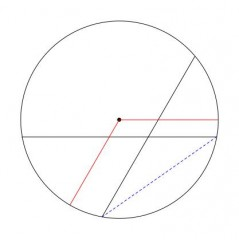# Secants BE and CF intersect at point D inside A . What is the measure of <CDE?

The measure of the angle at a point on the circle will be half the central angle which has the same arc endpoints.
Angle CEB = 1/2CAB = 25°
Angle ECF = 1/2EAF = 35°
CDE will be 180° - 25° - 35° = 120°

The angle of interest measures 120 degrees.

The figure below is drawn with the angle measures corresponding to those given. The red central angle is drawn as 120 degrees. You will note that its sides appear to be parallel to those of angle CDE.thanked the writer.